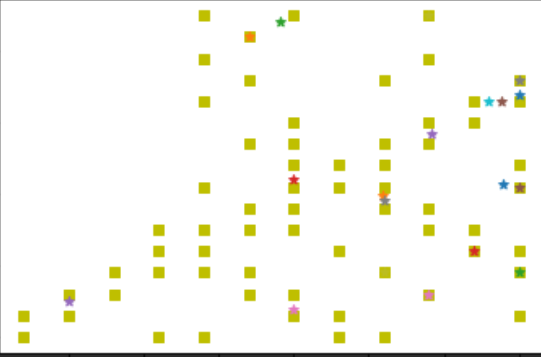# How to write a 2d image into a vtk file?

I use matplotlib to draw my results, and it looks like:Is there any way to write them into a .vtk file and looks like a 3D graph?

In Slicer you can plot data like this:

# Get a volume from SampleData and compute its histogram

import SampleData
import numpy as np
histogram = np.histogram(arrayFromVolume(volumeNode), bins=50)

chartNode = slicer.util.plot(histogram, xColumnIndex = 1)
chartNode.SetYAxisRangeAuto(False)
chartNode.SetYAxisRange(0, 4e5)

Hope it helps

Mauro

1 Like

you mean something like this?
then you can use `vtkImageActor ` class and save its input as vtk.

(code for the above):

``````from vedo import Picture, show
pic.show(bg2="green9").close()

# Another option:
# import numpy as np
# from vedo.pyplot import plot
# data = np.random.rand(200,2)
# plot(data, marker='s', mc="green5", lw=0).show()
``````
1 Like

Is there a way to remove background? And let squares and stars be like 3D objects( give them a thick)

but then you need to recreate the plot, for this you may use a `vtkGlyph` object.

`vedo` has this functionality, plus you can control the plot aspect ratio:

``````import numpy as np
from vedo.pyplot import plot
from vedo import Cube, Star
data = np.random.rand(200,2)
m = Cube().scale(0.025)
p = plot(data, marker=m, mc="green5", lw=0, aspect=16/9)
s = Star().scale(0.03).extrude(0.1)
p+= plot(data[:10], marker=s, mc="red5", lw=0, like=p)
p.unpack(1).lighting("default")
p.show()
``````

Thanks so much!
Btw, can I write .vtk file through matplotlib? cuz I draw images above through matplotlib, want to figure out if there’s a way to write 3D file at the same time

1 Like